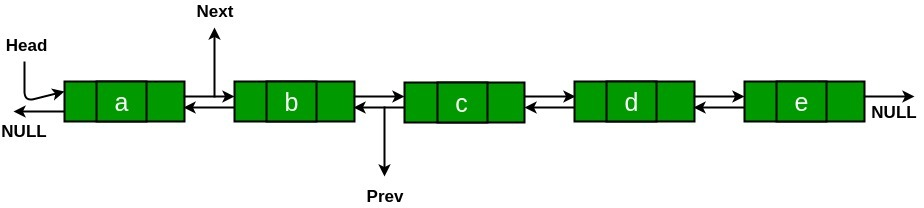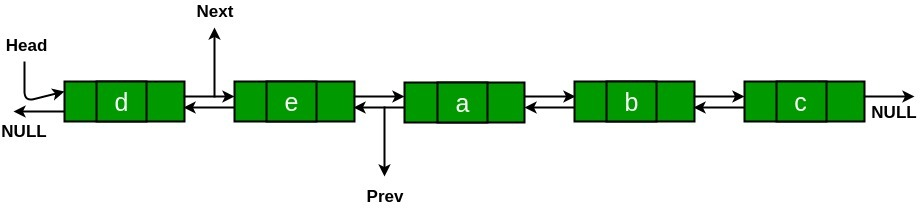Clockwise rotation of Doubly Linked List by N places

• Difficulty Level : Hard
• Last Updated : 08 Jun, 2021

Given a doubly-linked list and an integer N, the task is to rotate the linked list clockwise by N nodes.
Examples:

Input: N = 2Output:Approach: To rotate the Doubly linked list first check whether the given N is greater than the length of the list or not. If N is greater than the size of the list then deduce it in the range of linked list size by taking modulo with the length of the list. After that subtract the value of N from the length of the list. Now the problem reduces to the counter-clockwise rotation of a doubly-linked list by N places.

• Change the next of the last node to point the Head node.

• Change the prev of the Head node to point the last node.

• Change the value of the Head_ref to be the next of the Nth node.

• Change the value of next of the Nth Node to be NULL.

• Finally, make the prev of Head node to point to NULL.

Below is the implementation of the above approach:


Output: